# DiffEqBiological Tutorial III: Steady-States and Bifurcations

##### Torkel Loman and Samuel Isaacson

Several types of steady state analysis can be performed for networks defined with DiffEqBiological by utilizing homotopy continuation. This allows for finding the steady states and bifurcations within a large class of systems. In this tutorial we'll go through several examples of using this functionality.

using DiffEqBiological, Plots
gr(); default(fmt = :png);


### Steady states and stability of a biochemical reaction network.

Bistable switches are well known biological motifs, characterised by the presence of two different stable steady states.

bistable_switch = @reaction_network begin
d,    (X,Y) → ∅
hillR(Y,v1,K1,n1), ∅ → X
hillR(X,v2,K2,n2), ∅ → Y
end d v1 K1 n1 v2 K2 n2
d = 0.01;
v1 = 1.5; K1 = 30; n1 = 3;
v2 = 1.; K2 = 30; n2 = 3;
bistable_switch_p = [d, v1 ,K1, n1, v2, K2, n2];


The steady states can be found using the steady_states function (which takes a reaction network and a set of parameter values as input). The stability of these steady states can be found using the stability function.

ss = steady_states(bistable_switch, bistable_switch_p)

3-element Array{Array{Float64,1},1}:
[31.322504001213243, 46.769050724087236]
[3.970283396636649, 99.76874280256095]
[149.9972223365578, 0.7936945352275889]

stability(ss,bistable_switch, bistable_switch_p)

3-element Array{Bool,1}:
0
1
1


Since the equilibration methodology is based on homotopy continuation, it is not able to handle systems with non-integer exponents, or non polynomial reaction rates. Neither of the following two systems will work.

This system contains a non-integer exponent:

rn1 = @reaction_network begin
p, ∅ → X
hill(X,v,K,n), X → ∅
end p v K n
p1 = [1.,2.5,1.5,1.5]

ERROR: MethodError: no method matching ^(::DynamicPolynomials.PolyVar{true}, ::Float64)
Closest candidates are:
^(!Matched::Missing, ::Number) at missing.jl:94
^(!Matched::Float64, ::Float64) at math.jl:781
^(!Matched::Irrational{:ℯ}, ::Number) at mathconstants.jl:91
...


This system contains a logarithmic reaction rate:

rn2 = @reaction_network begin
p, ∅ → X
log(X), X → ∅
end p
p2 = [1.]

ERROR: This reaction network does not correspond to a polynomial system. Some of the reaction rate must contain non polynomial terms.


### Bifurcation diagrams for biochemical reaction networks

Bifurcation diagrams illustrate how the steady states of a system depend on one or more parameters. They can be computed with the bifurcations function. It takes the same arguments as steady_states, with the addition of the parameter one wants to vary, and an interval over which to vary it:

bif = bifurcations(bistable_switch, bistable_switch_p, :v1, (.1,5.))
plot(bif,ylabel="[X]",label="")
plot!([[],[]],color=[:blue :red],label = ["Stable" "Unstable"])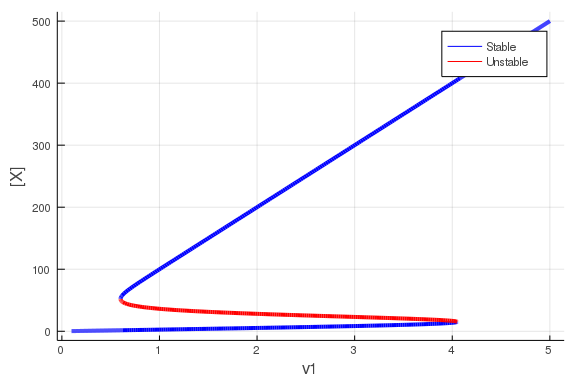The values for the second variable in the system can also be displayed, by giving that as an additional input to plot (it is the second argument, directly after the bifurcation diagram object):

plot(bif,2,ylabel="[Y]")
plot!([[],[]],color=[:blue :red],label = ["Stable" "Unstable"])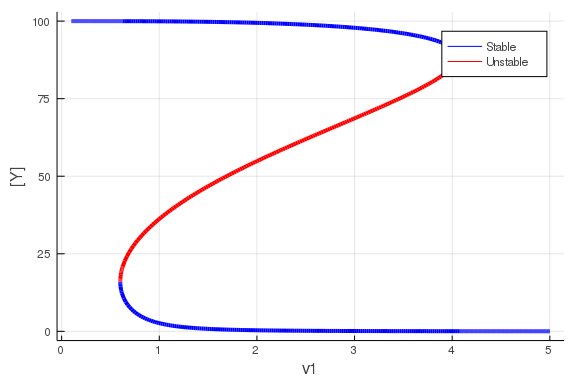The plot function also accepts all other arguments which the Plots.jl plot function accepts.

bif = bifurcations(bistable_switch, bistable_switch_p,:v1,(.1,10.))
plot!([[],[]],color=[:blue :red],label = ["Stable" "Unstable"])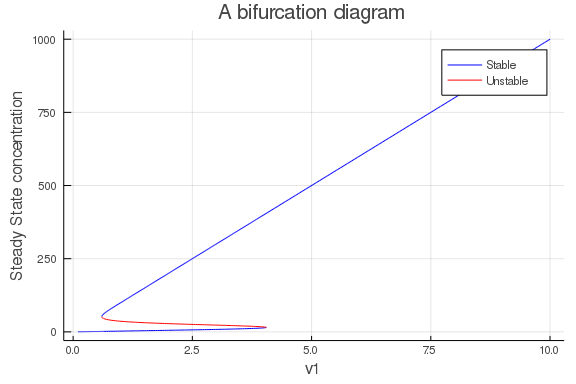Certain parameters, like n1, cannot be sensibly varied over a continuous interval. Instead, a discrete bifurcation diagram can be calculated with the bifurcation_grid function. Instead of an interval, the last argument is a range of numbers:

bif = bifurcation_grid(bistable_switch, bistable_switch_p,:n1,1.:5.)
plot(bif)
scatter!([[],[]],color=[:blue :red],label = ["Stable" "Unstable"])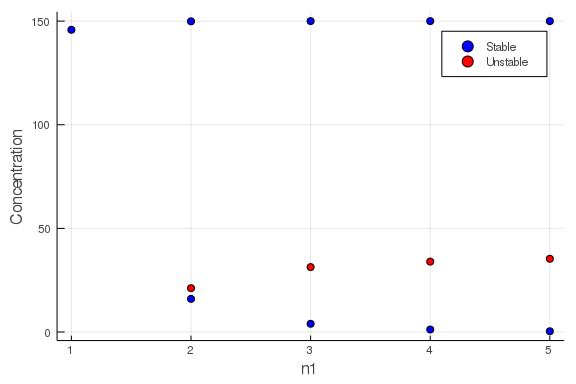### Bifurcation diagrams over two dimensions

In addition to the bifurcation diagrams illustrated above, where only a single variable is varied, it is also possible to investigate the steady state properties of s system as two different parameters are varied. Due to the nature of the underlying bifurcation algorithm it is not possible to continuously vary both parameters. Instead, a set of discrete values are selected for the first parameter, and a continuous interval for the second. Next, for each discrete value of the first parameter, a normal bifurcation diagram is created over the interval given for the second parameter.

bif = bifurcation_grid_diagram(bistable_switch, bistable_switch_p,:n1,0.:4.,:v1,(.1,5.))
plot(bif)
plot!([[],[]],color=[:blue :red],label = ["Stable" "Unstable"])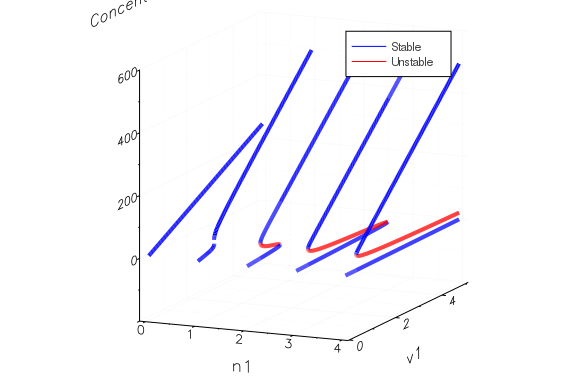In the single variable case we could use a bifurcation_grid to investigate the behavior of a parameter which could only attain discrete values. In the same way, if we are interested in two parameters, both of which require integer values, we can use bifrucation_grid_2d. In our case, this is required if we want to vary both the parameters n1 and n2:

bif = bifurcation_grid_2d(bistable_switch, bistable_switch_p,:n1,1.:3.,:n2,1.:10.)
plot(bif)
scatter!([[],[]],color=[:blue :red],label = ["Stable" "Unstable"])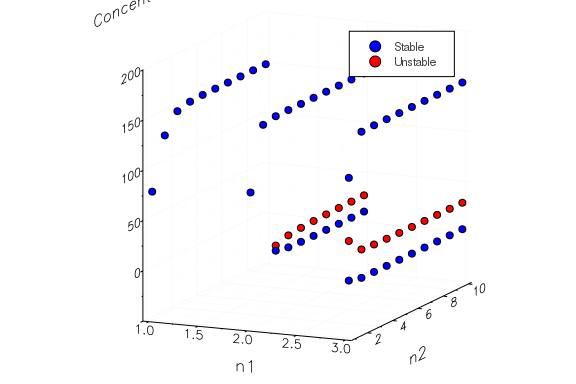### The Brusselator

The Brusselator is a well know reaction network, which may or may not oscillate, depending on parameter values.

brusselator = @reaction_network begin
A, ∅ → X
1, 2X + Y → 3X
B, X → Y
1, X → ∅
end A B;
A = 0.5; B = 4.;
brusselator_p = [A, B];


The system has only one steady state, for $(X,Y)=(A,B/A)$ This fixed point becomes unstable when $B > 1+A^2$, leading to oscillations. Bifurcation diagrams can be used to determine the system's stability, and hence look for where oscillations might appear in the Brusselator:

bif = bifurcations(brusselator,brusselator_p,:B,(0.1,2.5))
plot(bif,2)
plot!([[],[],[],[]],color=[:blue :cyan :orange :red],label = ["Stable Real" "Stable Complex" "Unstable Complex" "Unstable Real"])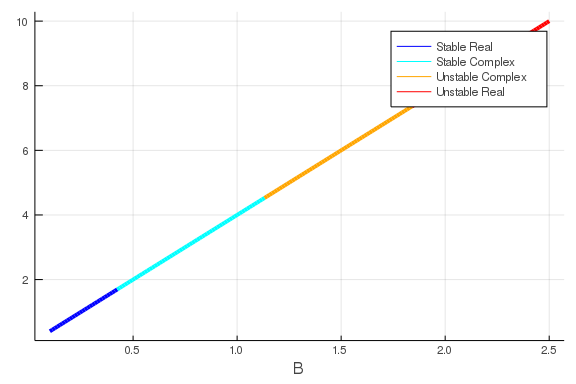Here red and yellow colors label unstable steady-states, while blue and cyan label stable steady-states. (In addition, yellow and cyan correspond to points where at least one eigenvalue of the Jacobian is imaginary, while red and blue correspond to points with real-valued eigenvalues.)

Given A=0.5, the point at which the system should become unstable is B=1.25. We can confirm this in the bifurcation diagram.

We can also investigate the behavior when we vary both parameters of the system:

bif = bifurcation_grid_diagram(brusselator,brusselator_p,:B,0.5:0.02:5.0,:A,(0.2,5.0))
plot(bif)
plot!([[],[],[],[]],color=[:blue :cyan :orange :red],label = ["Stable Real" "Stable Complex" "Unstable Complex" "Unstable Real"])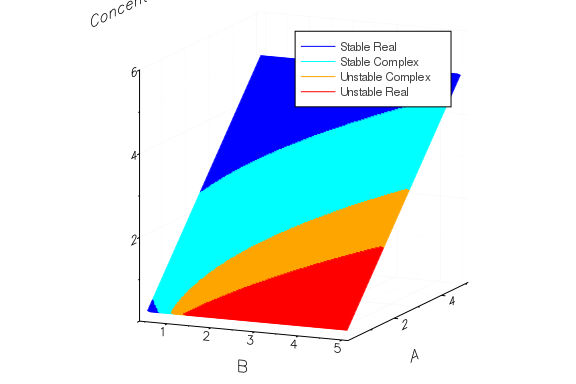## Getting Help

Have a question related to DiffEqBiological or this tutorial? Feel free to ask in the DifferentialEquations.jl Gitter. If you think you've found a bug in DiffEqBiological, or would like to request/discuss new functionality, feel free to open an issue on Github (but please check there is no related issue already open). If you've found a bug in this tutorial, or have a suggestion, feel free to open an issue on the DiffEqTutorials Github site. Or, submit a pull request to DiffEqTutorials updating the tutorial!

## Appendix

This tutorial is part of the DiffEqTutorials.jl repository, found at: https://github.com/JuliaDiffEq/DiffEqTutorials.jl

To locally run this tutorial, do the following commands:

using DiffEqTutorials
DiffEqTutorials.weave_file("models","04b-diffeqbio_III_steadystates.jmd")

Computer Information:

Julia Version 1.2.0
Commit c6da87ff4b (2019-08-20 00:03 UTC)
Platform Info:
OS: macOS (x86_64-apple-darwin18.6.0)
CPU: Intel(R) Core(TM) i7-6920HQ CPU @ 2.90GHz
WORD_SIZE: 64
LIBM: libopenlibm
LLVM: libLLVM-6.0.1 (ORCJIT, skylake)


Package Information:

Status ~/.julia/environments/v1.2/Project.toml
[6e4b80f9-dd63-53aa-95a3-0cdb28fa8baf] BenchmarkTools 0.4.3
[a93c6f00-e57d-5684-b7b6-d8193f3e46c0] DataFrames 0.19.4
[2b5f629d-d688-5b77-993f-72d75c75574e] DiffEqBase 6.3.4
[eb300fae-53e8-50a0-950c-e21f52c2b7e0] DiffEqBiological 4.0.1
[c894b116-72e5-5b58-be3c-e6d8d4ac2b12] DiffEqJump 6.2.2
[a077e3f3-b75c-5d7f-a0c6-6bc4c8ec64a9] DiffEqProblemLibrary 4.5.1
[6d1b261a-3be8-11e9-3f2f-0b112a9a8436] DiffEqTutorials 0.1.0
[0c46a032-eb83-5123-abaf-570d42b7fbaa] DifferentialEquations 6.8.0
[42fd0dbc-a981-5370-80f2-aaf504508153] IterativeSolvers 0.8.1
[23fbe1c1-3f47-55db-b15f-69d7ec21a316] Latexify 0.11.0
[54ca160b-1b9f-5127-a996-1867f4bc2a2c] ODEInterface 0.4.6
[47be7bcc-f1a6-5447-8b36-7eeeff7534fd] ORCA 0.3.0
[1dea7af3-3e70-54e6-95c3-0bf5283fa5ed] OrdinaryDiffEq 5.17.2
[f0f68f2c-4968-5e81-91da-67840de0976a] PlotlyJS 0.13.0
[91a5bcdd-55d7-5caf-9e0b-520d859cae80] Plots 0.27.0
[438e738f-606a-5dbb-bf0a-cddfbfd45ab0] PyCall 1.91.2
[d330b81b-6aea-500a-939a-2ce795aea3ee] PyPlot 2.8.2
[b4db0fb7-de2a-5028-82bf-5021f5cfa881] ReactionNetworkImporters 0.1.5
[d6f4376e-aef5-505a-96c1-9c027394607a] Markdown